Select Language

×

## What is Vibration Test?

vibration test is a testing method called “vibration test,” in which everyone considers the performance and quality of a product on a common basis and makes it possible to draw conclusions in a scientifically correct manner that enables objective recognition, and the method of testing for mechanical vibration is given.

Our surroundings are full of vibration-related problems.

For Example…

• When a car runs, the vibration generated by the engine is transmitted to each part of the car body. If the vibration is too severe, parts installed near the engine may fail. It may not happen. How can we identify it?
• If it does not immediately fail, is the life of the part shortened due to the engine vibration? How can it be guaranteed that the part can be used properly?
• The car shakes violently if the roads on which it is running are bumpy. Therefore, vibration is generated in each part of the car body. For this reason, music from the CD you were listening to now jumped. To prevent this, I tried to modify the CD player, but how should I check the effect of the modification?

The “vibration test system” is a device for realizing these in concrete terms.

## Mechanism of Vibration Test System

This section introduces the mechanism of the vibration test system.

### Electric Vibration Test System

Image your audio equipment. An amplifier amplifies an electric signal such as CD of a sound source, and vibrates the air with a speaker to transmit it as sound.When there is and the test conditions are registered and executed,Power amplifier It sends an electrical signal to the amplifiervibration test systemVibrate. However, the vibration controllerdiffers greatly from the CD deck and other devices, and the vibration pickup signals attached to the vibration test system are fed back to constantly monitor the vibration conditions and perform control to match the test conditions.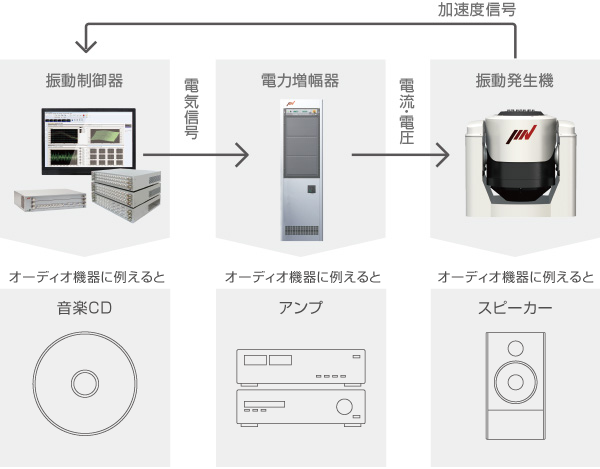### Vibration Test System

The principle of the vibration test system uses Fleming’s left-hand rule. When a current is applied to a conductor that crosses a magnetic field, a force is generated in the rectilinear direction, and the vibration table vibrates due to this force (exciting force). The following formula is used to calculate the force.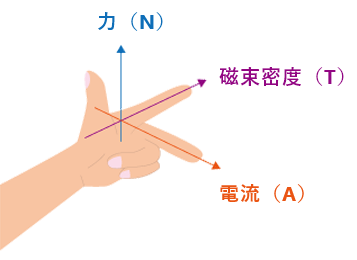「Fleming’s left-hand rule」• F：Force （N）
• B：Flux density （T）
• I：Electric current（A）
• L：Length of cable（ｍ）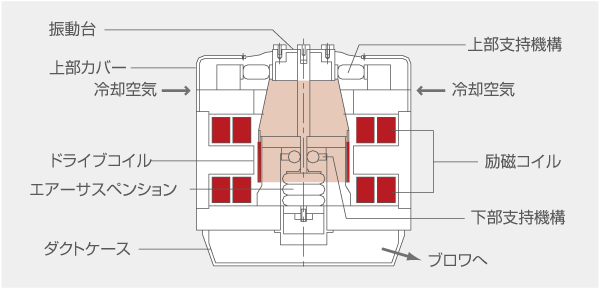Image of the inside of the vibration test system (air-cooled system)

•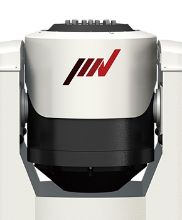vibration test system

•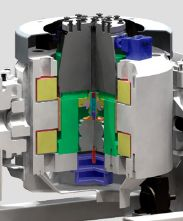Cross-sectional view of vibration test system

### Vibration Controller

Unfortunately, vibration of the same waveform cannot be generated even if the measured vibration data is directly connected to the power amplifier and excited. Depending on the characteristics of the power amplifier and vibration test system, the waveform may be quite different.The vibration controller reflects these characteristics and is used to automatically generate the target vibration. IMV’s vibration controller “K2” is a completely original in-house developed product, and we are constantly striving to improve user-friendliness and performance with the utmost care of our customers’ opinions. The latest “K2” not only allows you to perform various test methods without changing the hardware, but also enables you to easily set and execute complex tests by operating the software in full Japanese using a PC.

• #### Vibration Controller K2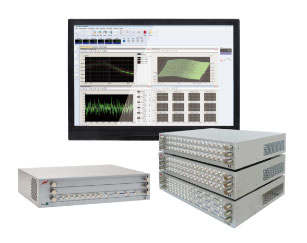• #### K2 Screen (Example)

Complex tests can also be easily set and executed.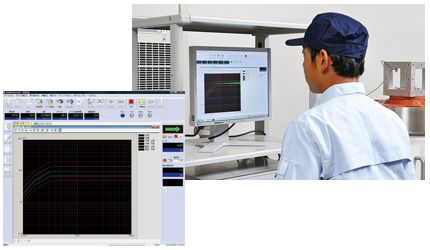### Power Amplifier

The purpose of the power amplifier is to supply power to the vibration test system.Based on a small signal from the vibration controller, much larger voltages and currents are created. The IMV’s power amplifiers use switching systems, with modules that are the industry’s most compact and highly efficient, contributing to space savings and energy savings.

•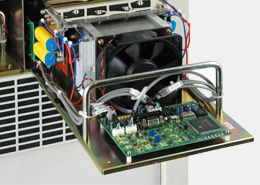Power Module SA-300

•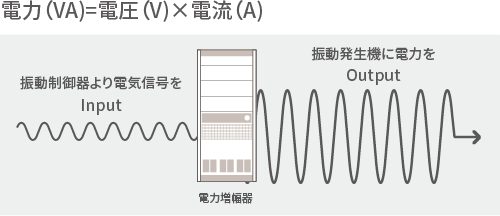image

## Type of Vibration Test（SINE, RANDOM, and SHOCK Tests）

This section explains the Sine vibration testing, random vibration test, and shock vibration test, as well as examples of their use.

### Sine Vibration Testing

Vibration with a fixed period can be given, or vibration can be given while sweeping a fixed period.Near structures with natural frequencies, this test is necessary (e.g. fans and motors)In addition, the natural frequency of the sample (= the weakness of the product) can be grasped by conducting a sweep test.

• #### Sweep Test

To continuously generate a sine with its frequency component while gradually changing the frequency from a certain frequency to a certain frequency. Waveform is continuous and changes over time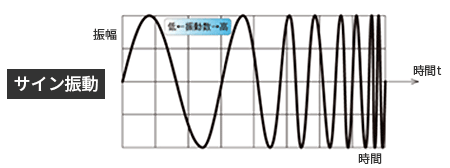• #### Spot Test

To generate a sine with a frequency component of a certain frequency continuously for a certain period of time. Similar waveforms continue (at the same frequency)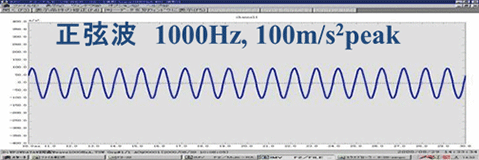### Random Vibration Test

This is an irregular waveform that cannot predict amplitude and frequency at any moment and can only be described from a statistical point of view.Since it is possible to vibrate with many frequency components at the same time as adding random vibration, vibration tests such as characteristic tests of resonance phenomena can be carried out in a short time.This is the vibration from the road surface when a car is running, or when a rocket breaks through the atmosphere and flies into outer space.
※ The vibration that actually occurs can be almost called random vibration.

### Shock Vibration Test

As the name goes, it reproduces the vibration when a desk was hit with a van or a car hit with a bon.
In the IMV controller, the horizontal axis is also used to give the oscillation which reproduces the measured wave form of the time-axis.

## What is Vibration Test Acceleration?

When performing a vibration test, the vibration and acceleration settings are generally used for the test. However, I often asked the question of how to think about acceleration.
For this reason, we will introduce the concept of acceleration in vibration tests.

If you want this content, you should know how to calculate and set the acceleration for the test you are going to start with.

### So let’s start with what acceleration is in the first place!

•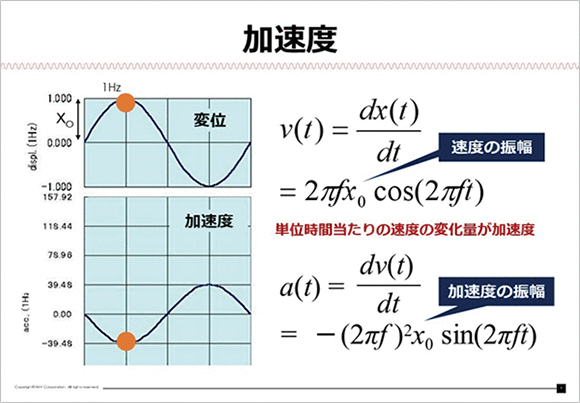• Unit
m/s2
What is acceleration?
This is an amount representing the amount of change in speed per unit time.
In other words, differentiating the speed by time results in acceleration. 1m/s2.If this is the case, it indicates that the m/s has changed in one second.
The graph below the figure above shows the acceleration graph of an object vibrating at 1Hz .

#### Formula For Calculating Acceleration

Equation a(t)=(dv(t))/dt=-(2πf)^2 x_0 sin(2πft) in the lower right is the general expression for velocity differentiated by time. Since the cos is differentiated, it changes to a negative sin. Since the position of the peak is shifted by an additional 90 degrees from the velocity, it matches the displacement graph. However, the sign is inverted because of the negative sin, which can be confirmed by viewing the graph.In other words, each value can be calculated by differentiating and integrating the displacement, velocity, and acceleration in time, and the proportional expression of the trigonometric function, which makes it possible to use the features of the periodic function as it is, making it very easy to handle in performing arithmetic processing.

#### Other and Precautions For Acceleration in Fibration Test

Calculate each maximum value of exciting force, acceleration, speed, and displacement from the test conditions, and confirm that these values are below the performance of the tester.

## Visual Vibration Test

This section introduces the actual movement of the vibration test system. This section mainly presents “1-axis excitation movement,” “3-axis simultaneous excitation movement,” and “2 types of actuator movement.”

## Type of Vibration Test System

IMV offers the following testers for a wide range of tests.